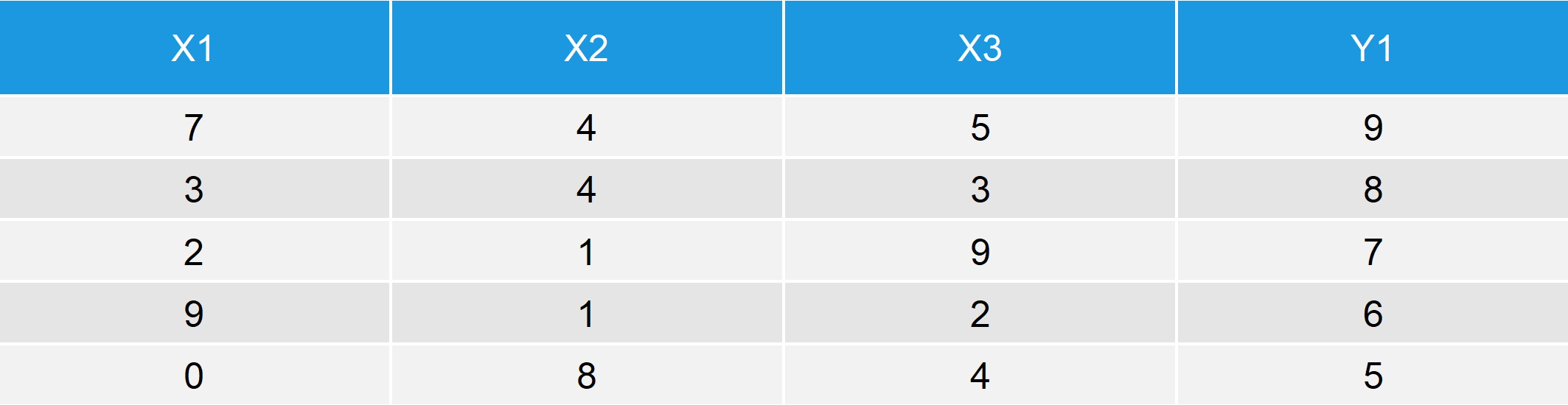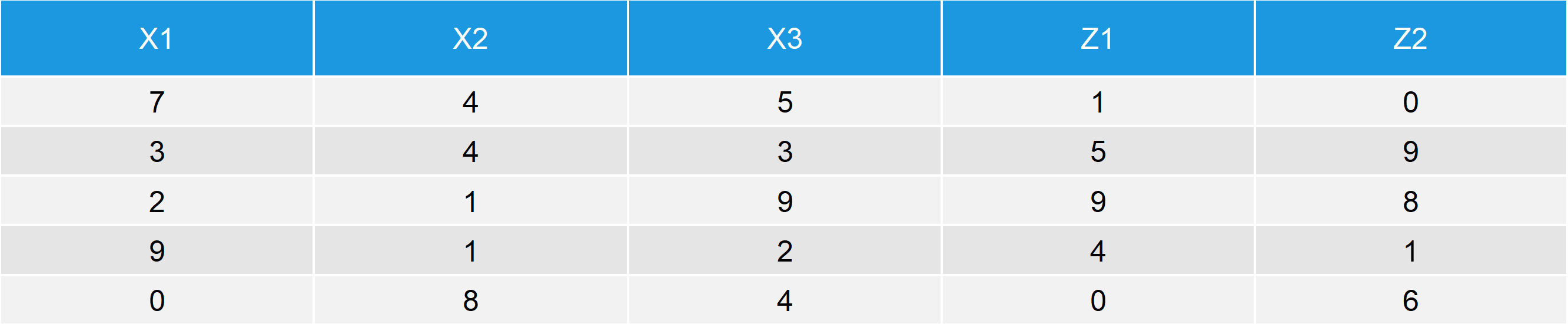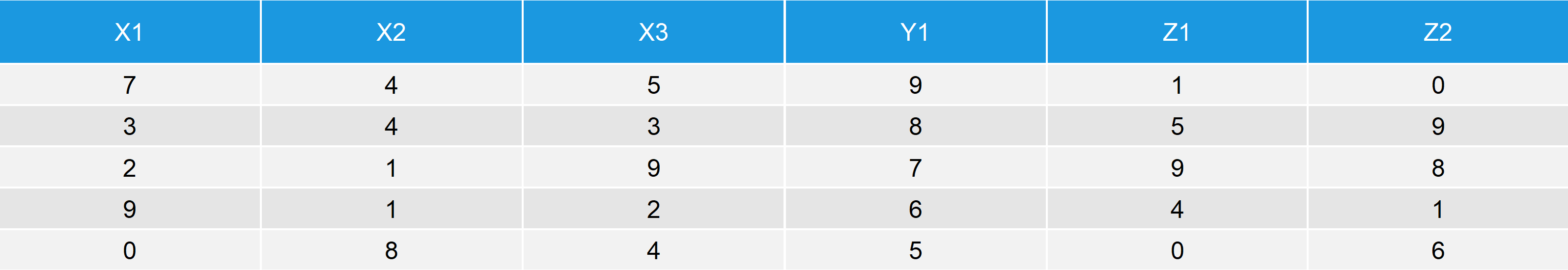# cbind R Command | 3 Example Codes (Data Frame, Vector & Multiple Columns)

Basic R Syntax:

`cbind(my_data, new_column)`

The name of the cbind R function stands for column-bind. The cbind function is used to combine vectors, matrices and/or data frames by columns. The code above, illustrates the basic syntax for cbind in R.

In the following article, I will show 3 examples for the usage of the cbind R command. If you want to know more about the cbind R function, keep reading.

## Example 1: cbind Vector to Data Frame

A popular way of using the cbind command in the R programming language is the combination of a vector and a data.frame. Before we can start with the first cbind example, let’s create an example data frame…

```data_1 <- data.frame(x1 = c(7, 3, 2, 9, 0),          # Column 1 of data frame
x2 = c(4, 4, 1, 1, 8),          # Column 2 of data frame
x3 = c(5, 3, 9, 2, 4))          # Column 3 of data frame```

…and an example vector / column:

`y1 <- c(9, 8, 7, 6, 5)                               # Create vector`

Now, we can cbind this vector as a new column to our example data frame:

```data_new1 <- cbind(data_1, y1)                       # cbind vector to data frame
data_new1                                            # Print data to RStudio console```Table 1: New Data Table after Column Binding a Vector to a Data Frame.

Table 1 illustrates the RStudio output of the cbind command: The first three columns of our new data matrix are identical to our original data frame data_1 and the fourth column is identical to the vector Y1.

## Example 2: cbind Two Data Frames in R Programming

The cbind command can be applied to two data frames in the same manner. First, let’s create a second data frame and cbind it to data_1 (the data table that we already used in Example 1):

```data_2 <- data.frame(z1 = c(1, 5, 9, 4, 0),          # Column 1 of data frame 2
z2 = c(0, 9, 8, 1, 6))          # Column 2 of data frame 2```

Now, let’s column bind these two data frames. We can use basically the same R code as in the cbind Example 1:

```data_new2 <- cbind(data_1, data_2)                   # cbind two data frames in R
data_new2                                            # Print data to RStudio console```Table 2: Output after Using cbind for Two Data Frames.

Similar to Example 1, the first three columns consist of data_1 and the last two columns of the output consist of data_2.

Note: Both data frames have the same column length. The cbind R function cannot be used, in case that the number of rows of the two data frames differs.

## Example 3: R cbind Multiple Columns

So far, we have applied cbind only in order to merge two data objects: In Example 1 to a data frame and a vector; in Example 2 to two data frames. However, the cbind function can also be applied to cbind multiple columns and data objects. For the following example, I’m using the data frame data_1; the vector Y1; and the data frame data_2 (created in the previous two examples):

```data_new3 <- cbind(data_1, y1, data_2)               # cbind two data frames and vector
data_new3                                            # Print data to RStudio console```Table 3: Application of cbind to Multiple Columns in R.

The first 3 columns of data_new3 consist of data_1; the fourth column consists of the vector Y1; and the last two columns consist of data_2.

Exactly, as we wanted – perfect!

## Video: cbind and rbind Explained

More explanations on how to copy multiple columns from one file to another using the cbind function needed? Then I can recommend the following video of my YouTube Channel. In the video, I’m explaining the R code of this tutorial in more detail.

Subscribe to the Statistics Globe Newsletter

•L zb
February 13, 2022 9:53 am

Nice explanation!

•February 14, 2022 8:35 am